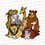# Elementary Techniques used in the IMO (International Mathematical Olympiad) - InvariantsThis post continues from both: Part 1 and Part 2

Part $3$

Applications

The first example is of a game. We will see how to apply the invariance principle.

Example 1: On a chessboard A and B play by turns to place black and white knights, respectively. Either player loses when he places a knight on a square attacked by a knight of the other colour or there are no free squares to place the knight on. If A starts, who has a winning strategy?

Solution: For those chess noobs like me, remark that a knight can only attack the $8$ squares shown in the figure below:tagtext

Intuitively, if each player can place a knight such that the squares that can be attacked by both knights do not overlap, then since a chessboard has an even number of cells, Player B can win, by in what people call, mirroring the first player.

Clearly a knight on a black square can only attack a knight on a white square and vice versa. So the colours of the squares that the knight attacks is invariant. Recall that a chessboard is a $8\times 8$, so if we "fold" the board along the middle, the $2$ "flaps" would be of opposing colour. For instance, let $a_{i,j}$ be the colour of the cell in the $ith$ row and $jth$ column of the chessboard. Then we have that $a_{4,2} = a_{4,7}$.

The above observation is key for us to see that the strategy for B is to do the same thing as A but symmetric to the center of the board. Basically, notice that if A can place a knight without being attacked by B's knights, the B can always place a "symmetric" knight without being attacked by A's knight due to the colour argument. Now given the way that knights attack, A can never place a knight that attacks the square where B played. Thus A loses in the end. □

To further train the skills of the reader in solving game-like problems (because we would soon be moving to colouring and other invariance, and I would select the IMO problems for demonstration), we will attempt one more example, this problem was in my training notes, and according to our trainer, was from Bulgaria 2001.

Example 2 Alice and Bob play by turns to write ones and zeroes in a list, from left to right. The game ends when each has written 2001 numbers. When the game ends the sequence of $0 ,1$ is interpreted in base $2$. Alice wins if that number can be written as a sum of two perfect squares and if otherwise, Bob wins. Who has a winning strategy?

Solution: We want a invariant that somehow involves squares. Well, preliminarily, just plain squares would remind me of $\pmod 4$. So ultimately one should think of Fermat's Christmas Theorem. Let's see how this property translates to a winning strategy.

Now suppose the number is $1100 \ldots 0$ where there is an even number of $0$, what can you observe? (Hint: consider $\pmod 4$) Yup, exactly! Since $4m$ can be written as a sum of $2$ squares iff $m$ can, we can perfectly well ignore the zeroes at the end. And notice that $11$ in base $2$ cannot be written as a sum of $2$ squares, so B wins! Generalising this argument, at any moment A writes a $1$, B just copies $A's$ move. In this way, if we ignore the zeores, the final number would end in $11$ which is congruent to $3 \pmod 4$ which by Fermat's Christmas Theorem, cannot be written as a sum of $2$ squares, so B wins.

Now, remember that $A$ is smart. She wants to avoid losing to $B$ in this way.

Can you complete the argument? Post your completed proof in the comments.

Announcement: I am extremely sorry but since I will be going overseas and the wifi there is unsteady, I will not be posting any more of these daily instalments until Friday, Singapore time.Note by Anqi Li
7 years, 5 months ago

This discussion board is a place to discuss our Daily Challenges and the math and science related to those challenges. Explanations are more than just a solution — they should explain the steps and thinking strategies that you used to obtain the solution. Comments should further the discussion of math and science.

When posting on Brilliant:

• Use the emojis to react to an explanation, whether you're congratulating a job well done , or just really confused .
• Ask specific questions about the challenge or the steps in somebody's explanation. Well-posed questions can add a lot to the discussion, but posting "I don't understand!" doesn't help anyone.
• Try to contribute something new to the discussion, whether it is an extension, generalization or other idea related to the challenge.

MarkdownAppears as
*italics* or _italics_ italics
**bold** or __bold__ bold
- bulleted- list
• bulleted
• list
1. numbered2. list
1. numbered
2. list
Note: you must add a full line of space before and after lists for them to show up correctly
paragraph 1paragraph 2

paragraph 1

paragraph 2

[example link](https://brilliant.org)example link
> This is a quote
This is a quote
    # I indented these lines
# 4 spaces, and now they show
# up as a code block.

print "hello world"
# I indented these lines
# 4 spaces, and now they show
# up as a code block.

print "hello world"
MathAppears as
Remember to wrap math in $$ ... $$ or $ ... $ to ensure proper formatting.
2 \times 3 $2 \times 3$
2^{34} $2^{34}$
a_{i-1} $a_{i-1}$
\frac{2}{3} $\frac{2}{3}$
\sqrt{2} $\sqrt{2}$
\sum_{i=1}^3 $\sum_{i=1}^3$
\sin \theta $\sin \theta$
\boxed{123} $\boxed{123}$

Sort by:

simo questions...

- 7 years, 5 months ago

Obviously

- 7 years, 5 months ago

simo?

- 6 years, 8 months ago